# Accelerating Scientific Code

author: Jacob Schreiber
contact: jmschreiber91@gmail.com

Oftentimes scientific research code is computationally expensive, as new data processing techniques are tested, or vast data sources are crunched. This problem can be exacerbated by graduate students and researchers who are stronger in theory than in efficient implementation. The ability to prototype quickly in Python can give the false impression that new algorithms are too computationally intensive for large scale work.

I am going to show some common optimization techniques for numerics code, based on my previous work with nanopore DNA sequencing. I will then show how this is fundamentally similar to regression trees and what lessons can be taken to make regression trees fast.

## Segmenting Nanopore Signals

The nanopore is a novel sequencing device proposed by David Deamer and Dan Branton over a decade ago. The gist is that a protein porin (the pore) is embedded in a cell membrane and immersed in a buffer fluid. When electrodes are put on both sides and voltage is applied, a constant ionic current flows through the pore. Single stranded DNA is strongly negatively charged, so when put in the system, will move towards the positive electrode. When the strand passes through the pore, the nucleotides impede the current. The limiting apperture is approximately four nucleotides long. The sequence specific fluctuations in ionic current can then be decoded back into 4mer sequence.

The specifics of how this work are not relevant, but the gist is that the electrodes read the current at 100,000 samples per second, and it can take between two and ten seconds for a strand to pass through the pore. This means a lot of data points, and so one must be careful with what algorithm they choose to use.

My work as part of the UCSC Nanopore Group was to write the computational framework to analyze this data at a higher level than the excel spreadsheets of manually picked mean values. This involved me writing PyPore to handle nanopore data, and yahmm as a hidden Markov model backend for my work.

In :
from PyPore.DataTypes import *
import seaborn as sns
%pylab inline
sns.set_style("white")
sns.despine()

file = File("14418007-s04.abf")

Couldn't import dot_parser, loading of dot files will not be possible.
Populating the interactive namespace from numpy and matplotlib

WARNING: pylab import has clobbered these variables: ['log', 'random', 'exp']
%matplotlib prevents importing * from pylab and numpy

<matplotlib.figure.Figure at 0x7f2f481a1190>

Now that we've loaded a file of data (12.5 minutes), lets take a look at some of the events.

In :
plt.figure( figsize=(15,5) )
file.plot( c='k', file_downsample=250, limits=(270,297.8) )
plt.show()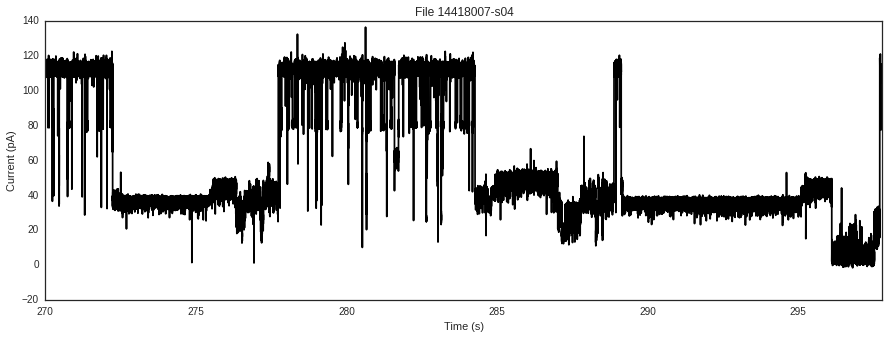We see a constant current of ~120 pA, with spikes downwards. Briefly, there are two types of events; enzyme bound and enzyme free events. Strands without an enzyme bound pass quickly through the porin and cannot be read, but strands with an enzyme present have their movement mediated by the enzyme and produce useful readings. Those are the longer events we will look at.

Lets use a simple rule parser to find these events; simply, they must be longer than a second, less than 90 pA, and never go below 0 pA. They are shown below in teal.

In :
file.parse()
plt.figure( figsize=(15,5) )
file.plot( c='k', color_events=True, file_downsample=250, limits=(270,297.8) )
plt.show()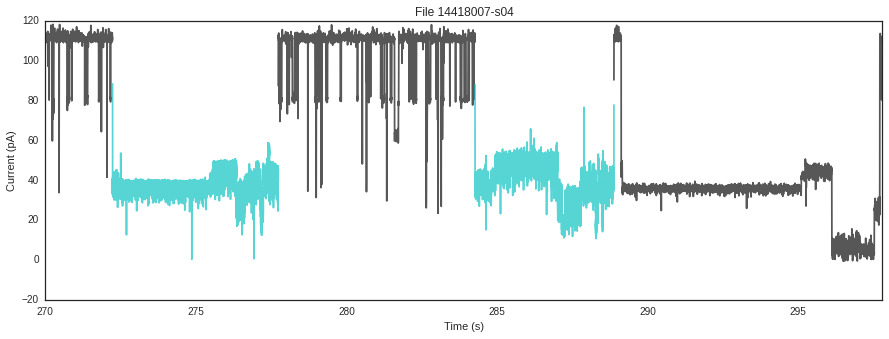Looking at the events from a distance, there doesn't seem to be much signal in them. Lets take a close look at some events, and we can begin to see some patterns.

In :
for event in file.events[1::10]:
event.filter()
plt.figure( figsize=(15,6) )
event.plot()
plt.show()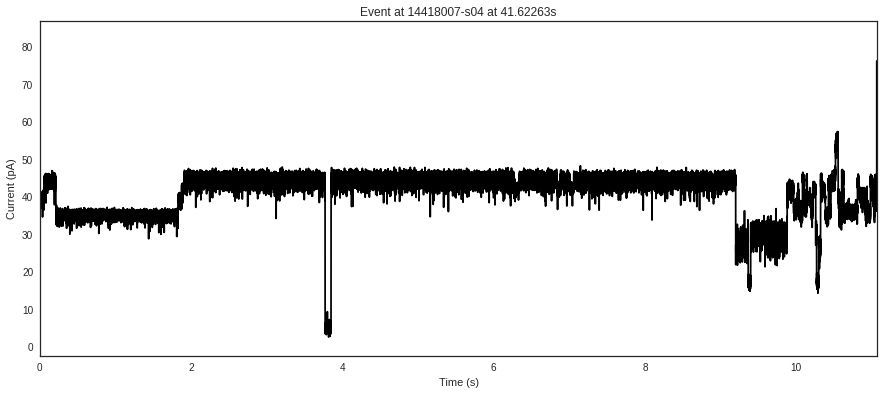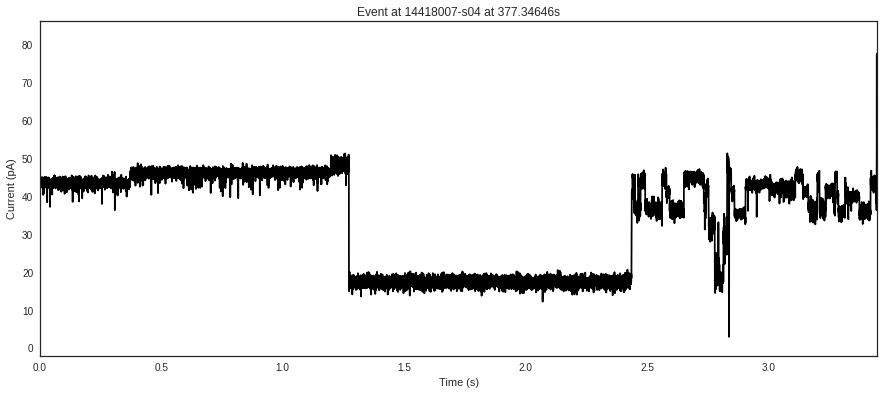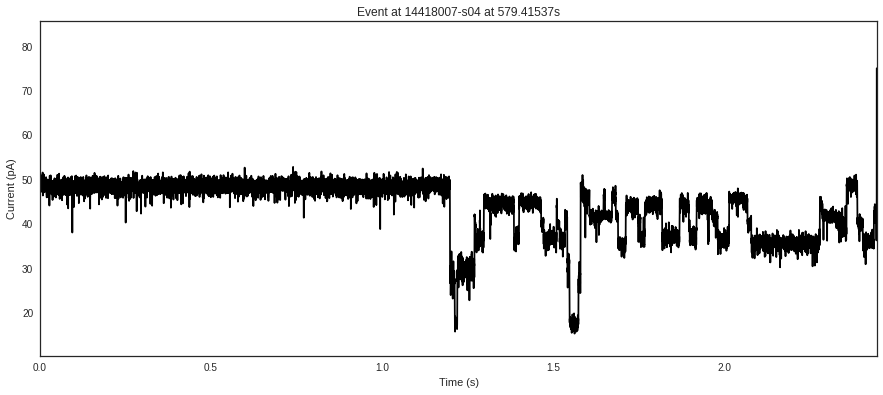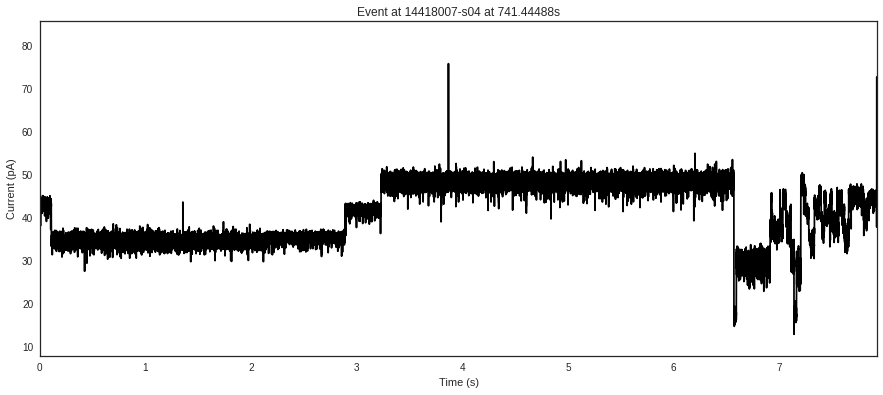We can see that there seems to be some vague pattern, which is made up of these 'blocks' of ionic current which form when a kmer is held in the limiting apperture of the pore before the enzyme ratchets the next nucleotide in. However, our task is not to derive or interpret this pattern, but to find a way to break up the signal into these blocks, which the next step will interpret.

Through your genius, you come up with the following idea, which is to recursively find 'the best split' in the data until it's properly segmented. You go through every point in the data, and ask 'what is the probabilistic gain in modelling the data as two Gaussians split at this point, versus one Gaussian at the entire point?' For every point you get a gain, and the best point is where you make the split. Here is the equation for ionic current $X$, start position $s$, end position $t$, and present position $i$.

\begin{equation} S(X[s:t], i) = \frac{P(X[s:i])P(X[i:t])}{P(X[s:t])} \end{equation}\begin{equation} P(X[s:t]) = \prod\limits_{j=s}^{t-1} P(X[j], mean(X[s:t]), std(X[s:t])) \end{equation}\begin{equation} P(x, \mu, \sigma) = -\frac{1}{\sigma\sqrt{2\pi}}e^{\frac{-(x-\mu)^{2}}{2\sigma^{2}}} \end{equation}

So lets go implement a finding a single split, and see how long it takes! Lets use synthetic data first.

In :
current = np.concatenate([ np.random.randn(100) + 25, np.random.randn(100) + 10 ])
plt.figure( figsize=(15,6) )
plt.plot(current, c='k')
plt.show()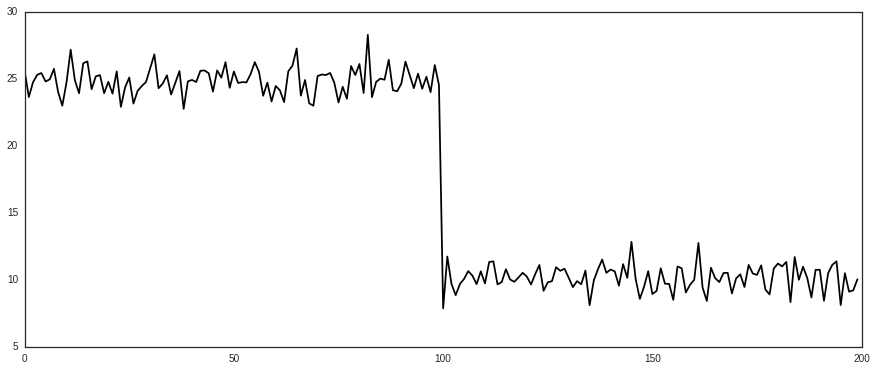In :
import numpy as np

def point_proba( x, mu, sigma ):
'''Return the probability of a point under the Gaussian equation'''
return -np.exp( -(x-mu) ** 2.0 / (2.0*sigma**2.0 ) ) / ( sigma * np.sqrt(2*np.pi) )

def segment_proba( X ):
'''Return the probability of a segment modelled by a Gaussian'''
return np.prod([ point_proba( X[i], X.mean(), X.std() ) for i in xrange(X.shape)])

def score( X, i ):
'''Return the score for a segment, given a split point'''
return ( segment_proba( X[:i] ) * segment_proba( X[i:] ) ) / segment_proba( X )

def best_split( X ):
'''Return the best split in a segment'''
best_pos = 0
best_gain = float("-inf")

for i in range( 2, X.shape-2 ):
gain = score( X, i )
if gain > best_gain:
best_pos = i
best_gain = gain

return best_pos

tic = time.time()
i = best_split( current )
print "Best split is at index {} of {}, and it took {}s".format( i, current.shape, time.time() - tic )

plt.figure( figsize=(15, 6) )
plt.plot( np.arange(i), current[:i], c='m' )
plt.plot( np.arange(i, current.shape), current[i:], c='k' )
plt.show()

Best split is at index 100 of 200, and it took 4.04704189301s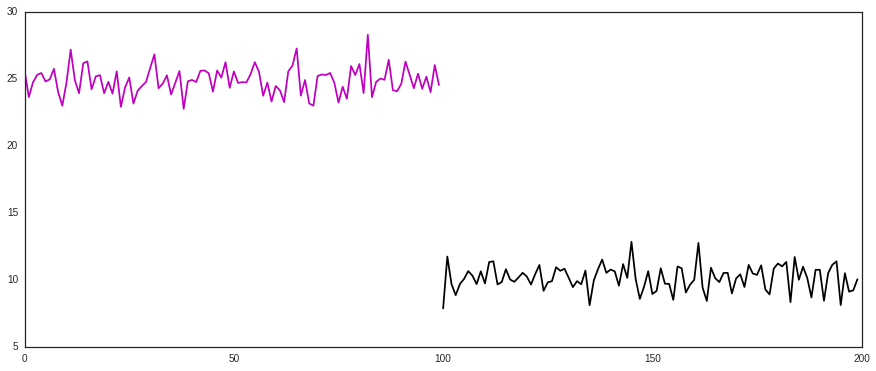Two things happen very quickly with these equations and the source data. The first is that it takes forever to run, on even a heavily subsampled portion of the data. The second is that it underflows very quickly and produces terrible results.

One suggestion may be to subsample until it goes quickly enough, but our goal here is quickly achieve exact results, not approximate our way out of the problem. The approximate results are also terrible.

The first thing to do, which is probably fairly obvious, is to switch from probabilities to log probabilities. We get the following equations:

\begin{equation} S(X[s:t], i) = ln P(X[s:i]) + ln P(X[i:t]) - ln P(X[s:t]) \end{equation}\begin{equation} ln P(X[s:t]) = \sum\limits_{j=s}^{t-1} ln P(X[j], mean(X[s:t]), std(X[s:t])) \end{equation}\begin{align} ln P(x, \mu, \sigma) = -ln(\sigma) -ln(\sqrt{2\pi}) - \frac{(x-\mu)^{2}}{2\sigma^{2}} \end{align}

These already look easier to work with.

In :
log_sqrt_2_pi = np.log( np.sqrt(2*np.pi) )

def point_log_proba( x, mu, sigma ):
'''Return the log probability of a point given a Gaussian distribution'''
return -np.log( sigma ) - log_sqrt_2_pi - (x - mu)**2.0 / (2.0 * sigma ** 2.0)

def segment_log_proba( X ):
'''Return the log probability of a segment'''
return np.sum([ point_log_proba( X[i], X.mean(), X.std() ) for i in xrange( X.shape ) ])

def score( X, i ):
'''Return the score for a segment, given a split point'''
return segment_log_proba( X[:i] ) + segment_log_proba( X[i:] ) - segment_log_proba( X )

tic = time.time()
i = best_split( current )
print "Best split is at index {} of {}, and it took {}s".format( i, current.shape, time.time() - tic )

plt.figure( figsize=(15, 6) )
plt.plot( np.arange(i), current[:i], c='m' )
plt.plot( np.arange(i, current.shape), current[i:], c='k' )
plt.show()

Best split is at index 100 of 200, and it took 2.6839838028sConverting to log space doesn't seem to make it any faster, but it does solve the underflow issue we encountered while making the equations a little eaiser to understand while preserving both the exact split position and likelihood ratio, should we desire it.

Now lets turn to making this faster. The nanopore samples at a rate of 100,000 samples per second, so taking 2 seconds to segment 200 points is infeasible. The first obvious optimization is to cache the mean and standard deviation calculated in segment_log_proba. The mean and standard deviation stay the same for each point in the segment, so we only need to calculate that once at the beginning, and pass it in each time.

In :
def segment_log_proba( X ):
'''Return the log probability of a segment'''
mean = X.mean()
std = X.std()
return np.sum([ point_log_proba( X[i], mean, std ) for i in xrange( X.shape ) ])

tic = time.time()
i = best_split( current )
print "Best split is at index {} of {}, and it took {}s".format( i, current.shape, time.time() - tic )

plt.figure( figsize=(15, 6) )
plt.plot( np.arange(i), current[:i], c='m' )
plt.plot( np.arange(i, current.shape), current[i:], c='k' )
plt.show()

Best split is at index 100 of 200, and it took 0.402736186981sThat worked surprisingly well! Off the bat, we already have almost a 10x speed increase. This makes sense, because instead of calculating means and standard deviations of arrays $3n^{2}$ times, we only calculate it $3n$ times now, since we call segment_log_proba once for each point when finding the best point to split at.

Lets get rid of some useless calculations for our next optimization. Previously, we defined:

\begin{equation} S(X[s:t], i) = ln P(X[s:i]) + ln P(X[i:t]) - ln P(X[s:t]) \end{equation}

but we know that $ln P(X[s:t])$ is a constant, and so won't actually affect finding the best split. We can either cache this result by calculating it once total, or get rid of it, since it's simply scaling a vector we're trying to find the argmax of. Lets define the new score function to be the following:

\begin{equation} S(X[s:t], i) = ln P(X[s:i]) + ln P(X[i:t]) \end{equation}

In :
def score( X, i ):
'''Return the score for a segment, given a split point'''
return segment_log_proba( X[:i] ) + segment_log_proba( X[i:] )

tic = time.time()
i = best_split( current )
print "Best split is at index {} of {}, and it took {}s".format( i, current.shape, time.time() - tic )

plt.figure( figsize=(15, 6) )
plt.plot( np.arange(i), current[:i], c='m' )
plt.plot( np.arange(i, current.shape), current[i:], c='k' )
plt.show()

Best split is at index 100 of 200, and it took 0.176352024078sThis seems to help a little bit, but does not remove the full one third of calculations because the computer was already caching this value.

Our next optimization is to combine point_log_proba and segment_log_proba to try to remove as many overlapping calculations as possible.

\begin{equation} ln P(X[s:t]) = \sum\limits_{j=s}^{t-1} ln P(X[j], mean(X[s:t]), std(X[s:t])) \end{equation}\begin{equation} ln P(x, \mu, \sigma) = -ln(\sigma) -ln(\sqrt{2\pi}) - \frac{(x-\mu)^{2}}{2\sigma^{2}} \end{equation}

We can combine these into a single equation with the following:

\begin{align} ln P(X[s:t]) &= \sum\limits_{j=s}^{t-1} -ln(\sigma) -ln(\sqrt{2\pi}) - \frac{(X[j]-\mu)^{2}}{2\sigma^{2}} \\ &= -(t-s) ln (\sigma) -(t-s) ln( \sqrt{2\pi} ) - \sum\limits_{j=s}^{t-1} \frac{(X[j]-\mu)^{2}}{2\sigma^{2}} \\ &= (t-s)(-\frac{1}{2} ln(2\pi) - ln(\sigma) ) + \frac{1}{2} \sum\limits_{j=s}^{t-1} \frac{(X[j]-\mu)^{2}}{2\sigma^{2}} \end{align}

We factor out the constants, which happen to be constant log functions, and only calculate them once, while only summing over the relevant, simpler, part.

In :
def segment_log_proba( X ):
'''Return the log probability of a segment'''
n = X.shape
mu = X.mean()
sigma = X.std()

return n*(-0.5*log_sqrt_2_pi - np.log(sigma)) + 0.5*np.sum([ (x - mu)**2.0 / (2.0*sigma**2.0) for x in X])

tic = time.time()
i = best_split( current )
print "Best split is at index {} of {}, and it took {}s".format( i, current.shape, time.time() - tic )

plt.figure( figsize=(15, 6) )
plt.plot( np.arange(i), current[:i], c='m' )
plt.plot( np.arange(i, current.shape), current[i:], c='k' )
plt.show()

Best split is at index 100 of 200, and it took 0.116543054581sThat helped a bit. But we're still not seeing big improvements here. Lets try simplifying it even further. Since mu and sigma are MLE estimates which we derived from the segment we're considering, by definition $\sum\limits_{j=s}^{t-1} \frac{(x-\mu)^{2}}{2\sigma^{2}}$ must be equal to one. So lets just remove it from our equation. This also removes the need to calculate the mean in the first place.

We can now simplify our segment_log_proba score to the following:

\begin{equation} ln P( X[s:t] ) = n (\frac{1}{2} ln(2\pi) - ln(\sigma) - 0.5) \end{equation}
In :
ln_2_pi = np.log(2*np.pi)

def segment_log_proba( X ):
'''Return the log probability of a segment'''
n = X.shape
sigma = X.std()

return n * (-0.5*ln_2_pi - np.log(sigma) - 0.5 )

tic = time.time()
i = best_split( current )
print "Best split is at index {} of {}, and it took {}s".format( i, current.shape, time.time() - tic )

plt.figure( figsize=(15, 6) )
plt.plot( np.arange(i), current[:i], c='m' )
plt.plot( np.arange(i, current.shape), current[i:], c='k' )
plt.show()

Best split is at index 100 of 200, and it took 0.0176467895508sAnother 10 fold improvement. This is good.

Lets collapse this down to just the score function then. Previously we had:

\begin{equation} S(X[s:t], i) = ln P(X[s:i]) + ln P(X[i:t]) \end{equation}

now we have:

\begin{align} S( X[s:t], i ) &= (i-s)(\frac{1}{2} ln(2\pi) - ln(\sigma_{s:i}) - 0.5) + (t-i)(\frac{1}{2} ln(2\pi) - ln(\sigma_{i:t}) - 0.5) \\ &= (t-s)(\frac{1}{2} ln (2\pi) - 0.5) - (i-s) ln(\sigma_{s:i}) - (t-i) ln(\sigma_{i:t}) \\ &= -(i-s)ln(\sigma_{s:i}) - (t-i) ln(\sigma_{i:t}) \end{align}
In :
def score( X, i ):
'''Return the score for a segment, given a split point'''
n = X.shape
sigma_l, sigma_r = X[:i].std(), X[i:].std()

return -i*sigma_l - (n-i)*sigma_r

tic = time.time()
i = best_split( current )
print "Best split is at index {} of {}, and it took {}s".format( i, current.shape, time.time() - tic )

plt.figure( figsize=(15, 6) )
plt.plot( np.arange(i), current[:i], c='m' )
plt.plot( np.arange(i, current.shape), current[i:], c='k' )
plt.show()

Best split is at index 100 of 200, and it took 0.0167770385742sAt this point, some may be satisfied with being 100x faster than before and be done with it, especially since it seems that we've narrowed it down as much as possible. I mean, it's just a few numbers now, when before it was many numbers!

Looking at the code, the most compute intensive part is the calculation of the standard deviation. It is unlikely that you can get much faster while maintaining exact precision, so we'll move on to the next optimization, which is caching between splits. When we calculate the standard deviation, we repeat many computations because we've already seen regions before. Lets look at the variance equation:

\begin{equation} Var( X ) = E[X^{2}] - E[X]^{2} \end{equation}

We can cache both $E[X^{2}]$ and $E[X]^{2}$ by taking the cumulative sum of $X$ and $X^{2}$ once across the entire array. When we want the expectation of any subarray, that's equivalent to subtracting the cumulative sum at the end from the cumulative sum at the beginning. In this manner, we can cache standard deviation calculations between splits. Lets take a look:

In :
def best_split( X ):
'''Return the best split in a segment'''

c = np.cumsum( X )
c2 = np.cumsum( np.multiply(X,X) )

best_pos = 0
best_gain = float("-inf")

n = float(X.shape)

for i in range( 2, X.shape-2 ):
low_var = (c2[i] - c2) / i - ((c[i] - c) / i)**2.0
high_var = (c2[n-1] - c2[i]) / (n-i) - ((c[n-1] - c[i]) / (n-i))**2.0

gain = -i*low_var - (n-i)*high_var

if gain > best_gain:
best_pos = i
best_gain = gain

return best_pos

tic = time.time()
i = best_split( current )
print "Best split is at index {} of {}, and it took {}s".format( i, current.shape, time.time() - tic )

plt.figure( figsize=(15, 6) )
plt.plot( np.arange(i), current[:i], c='m' )
plt.plot( np.arange(i, current.shape), current[i:], c='k' )
plt.show()

Best split is at index 99 of 200, and it took 0.00107908248901s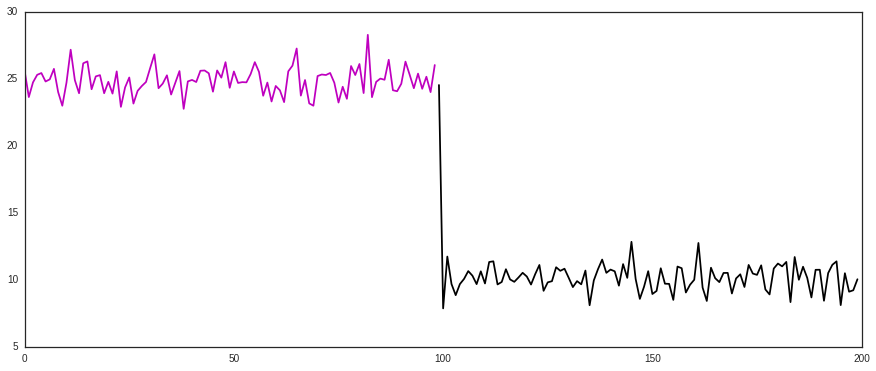This gives another 3x speedup. I have an off by one error somewhere in my code but it will give exact answers when that issue is resolved.

Keep in mind, we've achieved approximately a 300x speedup entirely in Python. No need to touch fancy JIT or cython magic. We've also significantly reduced the size of the code while providing a speedup, showing that we don't necessarily need more complex code to achieve speed--often times quite the opposite.

So, now lets write more code and use cython magic to see what we can get. In addition to cythonizing, we can collapse the variance equaion, since we weight by number of samples in the gain, but divide by number of samples in the variance calculation. This does not cause a significant speedup by itself, just reduces the size of the code.

In :
%load_ext Cython

In :
%%cython

cimport numpy
import numpy

from libc.stdlib cimport calloc, free
from libc.math cimport log

cpdef tuple best_split( double [:] X, int min_samples=2 ):
'''Find the best split, but Cython style'''

cdef int i, n = X.shape
cdef double* c = <double*> calloc(n, sizeof(double) )
cdef double* c2 = <double*> calloc(n, sizeof(double) )

c = X
c2 = X*X
for i in range(1, n):
c[i] = c[i-1] + X[i]
c2[i] = c2[i-1] + X[i]*X[i]

cdef double low_var, high_var, gain
cdef total_var
cdef double best_low_var, best_high_var
cdef double best_gain = -numpy.inf
cdef int best_pos = -1

total_var = c2[n-1] - c2 - (c[n-1] - c) ** 2.0 / n

for i in range( min_samples, n-min_samples ):
low_var = c2[i] - c2 - (c[i] - c) ** 2.0 / i
high_var = c2[n-1] - c2[i] - (c[n-1] - c[i]) ** 2.0 / (n-i)
gain = -low_var - high_var

if gain > best_gain:
best_pos = i
best_gain = gain
best_low_var = low_var
best_high_var = high_var

if best_pos == -1:
best_gain = -numpy.inf
else:
best_gain = log(best_low_var) + log(best_high_var) - log(total_var)

free(c)
free(c2)
return (best_pos, best_gain)

In :
tic = time.time()
i, gain = best_split( current )
print "Best split is at index {} of {}, and it took {}s".format( i, current.shape, time.time() - tic )

plt.figure( figsize=(15, 6) )
plt.plot( np.arange(i), current[:i], c='m' )
plt.plot( np.arange(i, current.shape), current[i:], c='k' )
plt.show()

Best split is at index 99 of 200, and it took 0.000130176544189sCythonizing the code at this point gives a ~11x speedup, while still preserving the exactness of the algorithm. Learning some cython is usually worth it so that you can turn compute intensive functions into faster functions. In this case, the main gains came from statically typing variables, using a C optimized loop instead of a Python loop, and storing the cumulative sum as a Cython buffer instead of a python object, allowing faster access.

We've been working a lot with this toy example, and we're getting down to the bare bones. Lets make sure we can properly see speed increases by returning to our real, nanopore data. Keep in mind that it used to take over 2 seconds to segment 200 points, and now lets look at an event with 1,106,597 samples.

In :
current = file.events.current

tic = time.time()
i, gain = best_split( current )
print "Best split is at index {} of {}, and it took {}s".format( i, current.shape, time.time() - tic )

plt.figure( figsize=(15, 6) )
plt.plot( np.arange(i), current[:i], c='m' )
plt.plot( np.arange(i, current.shape), current[i:], c='k' )
plt.show()

Best split is at index 920065 of 1106597, and it took 0.0405468940735s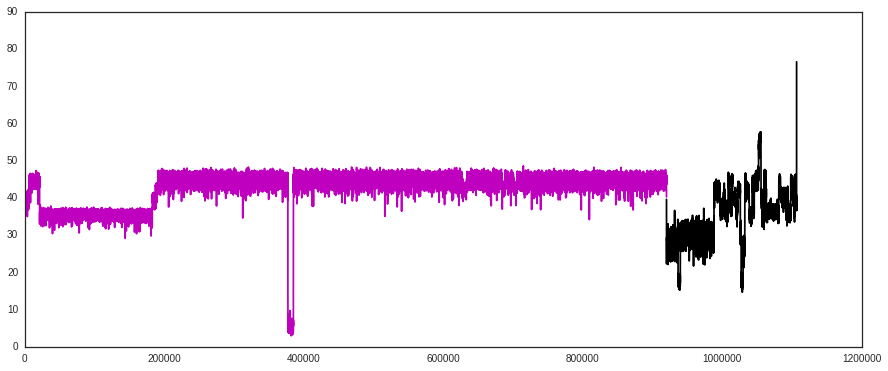0.07 seconds to segment that! It seems like this strategy may be feasible after all. After all, we're ~3,300x faster than before, now.

Lets simplify the gain calculation a little bit, by substituting in the two variances. We can cancel out many of the terms. In fact, if we weren't trying to calculate the exact gains to return, we could get rid of the cumulative value of $X^{2}$. The reason we want the exact gains is not because of needing it for a single split, but because we need a threshold for later to stop doing a recursive split.

In :
%%cython

cimport numpy
import numpy

from libc.stdlib cimport calloc, free
from libc.math cimport log

cpdef tuple best_split( double [:] X, int min_samples=2 ):
'''Find the best split, but Cython style'''

cdef int i, n = X.shape
cdef double* c = <double*> calloc(n, sizeof(double) )
cdef double* c2 = <double*> calloc(n, sizeof(double) )

c = X
c2 = X*X
for i in range(1, n):
c[i] = c[i-1] + X[i]
c2[i] = c2[i-1] + X[i]*X[i]

cdef double gain
cdef total_var
cdef double best_low_var, best_high_var
cdef double best_gain = -numpy.inf
cdef int best_pos = -1

for i in range( min_samples, n-min_samples ):
gain = (c[i] - c) ** 2.0 / i + (c[n-1] - c[i]) ** 2.0 / (n-i)

if gain > best_gain:
best_pos = i
best_gain = gain

total_var = c2[n-1] - c2 - (c[n-1] - c) ** 2.0 / n
best_high_var = c2[n-1] - c2[best_pos] - (c[n-1] - c[best_pos]) ** 2.0 / (n-best_pos)
best_low_var = c2[best_pos] - c2 - (c[best_pos] - c) ** 2.0 / best_pos

if best_pos == -1:
best_gain = -numpy.inf
else:
best_gain = log(best_low_var) + log(best_high_var) - log(total_var)

free(c)
free(c2)
return (best_pos, best_gain)

In :
tic = time.time()
i, gain = best_split( current )
print "Best split is at index {} of {}, and it took {}s".format( i, current.shape, time.time() - tic )

plt.figure( figsize=(15, 6) )
plt.plot( np.arange(i), current[:i], c='m' )
plt.plot( np.arange(i, current.shape), current[i:], c='k'